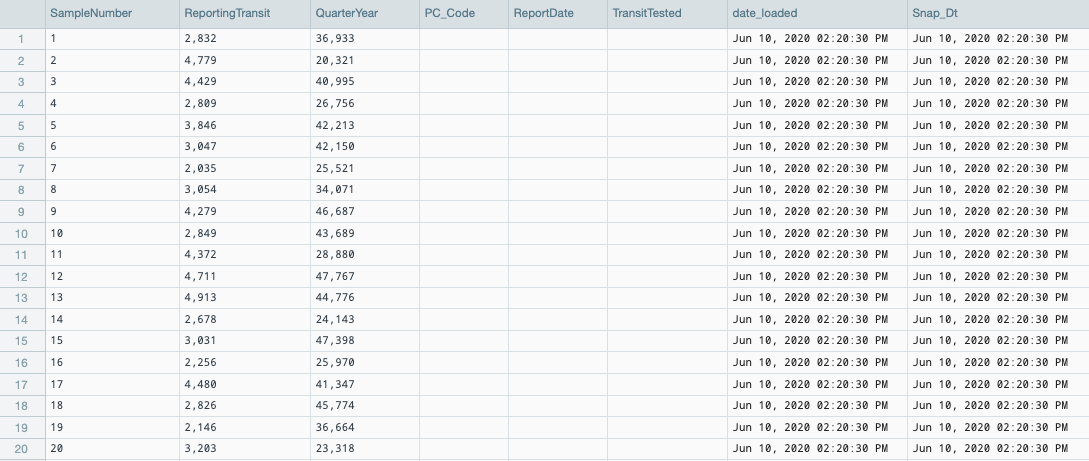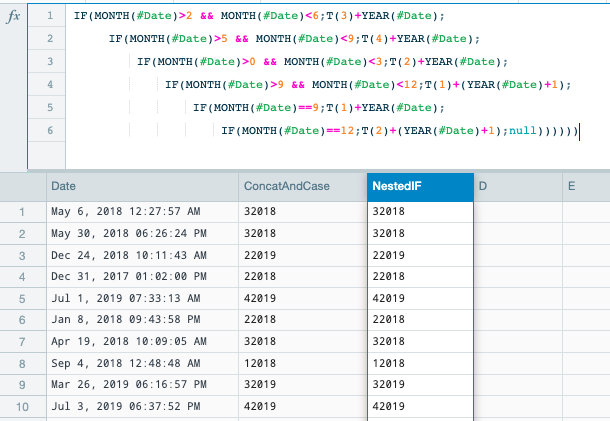•Hello Bhupinder.
I hope you are doing fine.

The conception of a Workbook does not intend manual data insertion. Usually one brings a dataset into Datameer via a FileUpload, an ImportJob or a DataLink and then loads this data into a Workbook for further analysis and transformation.

While it is possible to generate data in a Workbook without external sources, one can't create an empty column of a certain data type. First, it is required to generate value and then transform this value as needed.

In case I properly understand your use-case, you need to generate some data under a certain format. So, you could try the following approach.

• Create a new Workbook.
• In column A:
1. Add function EXPLODE_RANGE(1;20) - it creates 20 records of integers from 1 to 20.
2. Rename the columns to SampleNumber.
• Column B
1. Add function INT(RANDBETWEEN(2000;5000)) - it generates a random integer value between 2000 and 5000 for every record.
2. Rename the columns to ReportingTransit.
• Column C
1. Add function INT(RANDBETWEEN(20000;50000)) - it generates a random integer value between 20000 and 50000 for every record.
2. Rename the columns to QuarterYear.
• Columns D, E, F
1. Add function IF(#QuarterYear == 0; 25; null) - it generates null values for every record, as the condition always false. Note the type of the column will be INTEGER, as THEN part is an integer (25).
2. Rename the columns to PC_Code, ReportDate, and TransitTested accordingly.
• Columns G and H
1. Add function NOW() - it generates current time and date value for every record. The column type is DATE.
2. Rename the columns to date_loaded and Snap_Dt accordingly.

After these manipulations, you should get the following Sheet in the Workbook.However, I guess it is much easier to create the desired schema with sample records in a CSV file and ingest it into Datameer.

Please let me know if you will have any further questions.

•Thankyou for the code

QuarterYear should be dynamic usign the following logic from sql server

```(SELECT CAST(CASE WHEN month(getdate()) in (9,10,11) THEN 1  --12020 is availability date Oct/Nov/Dec 2019
WHEN month(getdate()) in (12,1,2) THEN 2 --22020 is availability date Jan/Feb/Mar 2020
WHEN month(getdate()) in (3,4,5) THEN 3  --32020 is availability date Apr/May/Jun 2020
WHEN month(getdate()) in (6,7,8) THEN 4  --42020 is availability date Jul/Aug/Sep 2020
END as Varchar(2)) + CAST(CASE WHEN month(getdate()) in (10,11,12) THEN Year(Getdate())+1 ELSE Year(Getdate()) END as varchar(4)))```

I have create the following formula seems complex and was if it could be simplified

CONCAT(T(CASE(0;month(NOW());9;1;10;1;11;1;12;2;1;2;2;2;3;3;4;3;5;3;6;4;7;4;8;4));T(CASE(YEAR(NOW());MONTH(NOW());10;YEAR(NOW())+1;11;YEAR(NOW())+1;12;YEAR(NOW())+1)))

•Hello Bhupinder.

In most of the cases, there is no good or bad formula for Datameer. The key is accuracy - in case the formula you created is 100% accurate and returns desired results, you are good to go.

I've represented the same logic as a set of nested IF functions and got the same results.

`IF(MONTH(#Date)>2 && MONTH(#Date)<6;T(3)+YEAR(#Date);   IF(MONTH(#Date)>5 && MONTH(#Date)<9;T(4)+YEAR(#Date);     IF(MONTH(#Date)>0 && MONTH(#Date)<3;T(2)+YEAR(#Date);       IF(MONTH(#Date)>9 && MONTH(#Date)<12;T(1)+(YEAR(#Date)+1);         IF(MONTH(#Date)==9;T(1)+YEAR(#Date);           IF(MONTH(#Date)==12;T(2)+(YEAR(#Date)+1);null))))))`•Thankyou kindly